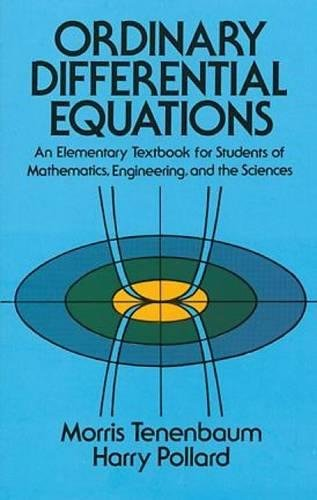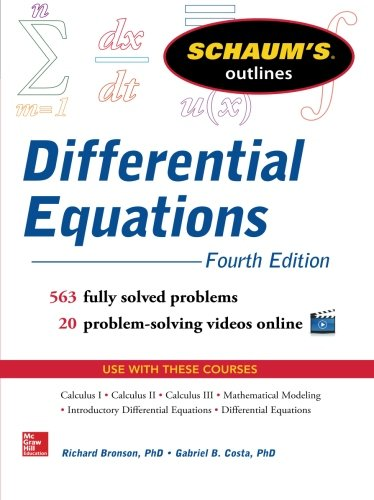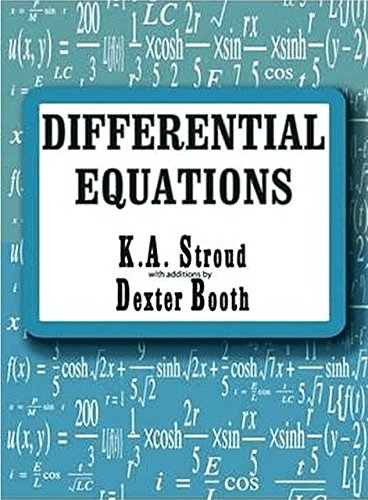Best Differential Equations books for applied mathematicians, physicists, and engineers

A differential equation is a mathematical equation which relates a function with one or more of its derivatives and that functions will satisfy the equations. During reading these book you will realize that math is really a fun when the core of the concepts is clear.

Here you will get some books about Differential Equations.

1. A First Course in Differential Equations with Modeling Applications

A First Course in Differential Equations with Modeling Applications is a great book for you to understand when to use certain concepts, equations, and tables. This is a very good introductory text into thermodynamics for undergraduate students. It contains lots of example problems in the text, a good mixture of sample problems and a good explanation of the topics to reinforce your understanding of the material.

It covers all topics to help you to prepare for the upcoming tests

• Introduction to differential equations
• First-order differential equations
• Modeling with first-order differential equations
• Higher-order differential equations
• Modeling with higher-order differential equations
• Series solutions of linear equations
• The Laplace transform
• Systems of linear first-order differential equations
• Numerical solutions of ordinary differential equations.

2. Ordinary Differential Equations (Dover Books on Mathematics)Author: Morris Tenenbaum,Harry Pollard
Published at: 01/10/1985
ISBN: 0486649407

Ordinary Differential Equations are recommended to anyone interested in learning deeper about differential equations, especially, engineering students. It gives you a plenty of examples and many end-of-chapter exercises which will help you to learn differential equations.

What you'll learn

• Why we use differential equations and how
• The theorem of existence and uniqueness
• The basic concepts of mathematical analysis needed to prove the theorem
• A complete and accurate proof of every logic.
• The relationship between variables and their derivatives.

Some important topics are

• Integrating factors
• Dilution and accretion problems
• The algebra of complex numbers
• The linearization of first-order systems
• Laplace Transforms
• Newton's Interpolation Formulas
• Picard's Method of Successive Approximations
• the Legendre Differential Equation
• Legendre Functions
• Legendre Polynomials
• The Bessel Differential Equation

3. Schaum's Outline of Differential Equations, 4th Edition (Schaum's Outlines)Author: Richard Bronson,Gabriel Costa
Published at: 12/03/2014
ISBN: 0071824855

This guide provides everything that you need to build confidence, skills, and knowledge for the highest score possible. This book is also a great book for your GRE preparation.  it provides you a different explanation about the method of solving the differential equation to reinforce the different methods of solving the differential equations.

Schaum's Outline of Differential Equations provides

• 563 fully solved problems
• Examples, and practice exercises to sharpen your problem-solving skills
• 30 detailed videos featuring Math instructors
• A concise explanation of all course concepts
• Covers first-order, second-order, and nth-order equations.

4. Differential Equations (Quick Study Academic)

This is for quick study. You can learn second-order nonhomogeneous linear differential equations, the method of undetermined coefficients, the variation of parameters
series solutions of linear equations, Laplace transform, numerical methods for solving DEQs, partial DEQs at a glance.

This includes

• Review of the indefinite integral
• Review of integration
• Basic definitions
• Classifying DEQs
• Initial-value problems
• Separable DEQs
• Exact equations
• Solving a first-order linear equations
• First-Order homogeneous equations
• Bernoulli equations
• Applications of the first-order DEQs
• Higher order linear DEQs
• Reduction of order
• Second-order homogeneous linear equations with constant coefficients
• Higher-order homogeneous linear equations with constant coefficients.

5. Differential Equations in 24 Hours: with Solutions and Historical Notes

Differential Equations in 24 hours is divided into 24 chapters labeled Hours. It will save your time by requiring a minimum amount of time. It contains many examples, exercises to improve your knowledge of understanding and help you to learn thing clearly.

This book gives you

• Differential equations in context with the physics
• Sufficient number of exercises and examples
• Solutions to all of the exercises
• Simple and practical physics problems
• The calculus used to solve problems
• Real applications in "Physics 101".

6. Partial Differential Equations with Fourier Series and Boundary Value Problems: Third Edition (Dover Books on Mathematics)

This is a very efficient book for undergraduates in mathematics, physics, engineering, and other fields who have completed a course in ordinary differential equations. By reading this book you will be able to know first-order equations, trigonometric series, PDEs in rectangular, polar, and spherical systems and associated eigenfunction expansions, Sturm-Liouville theory, the Fourier transform, Laplace/Hankel transforms for PDEs, grid-type numerical methods, sampling & discrete Fourier analysis, quantum mechanics and much more.

This book provides you

• A great transition from solving ordinary differential equations to solving partial differential equations
• Relevant derivations of core functions and equations like Bessel, Lagrange polynomials etc
• Clear explanation of Griffiths text
• Lots of tedious math writing out long series expansions
• Boundary value problems, including Fourier series
• An introduction to the partial differential equation.

7. Differential EquationsAuthor: K. A. Stroud,Dexter Booth
Published at: 10/01/2004
ISBN: 083113187X
Differential Equations offers you many practical examples to understand the topics from the root. It will be a very effective learning tool and reference for those who want to reinforce their basic understanding of mathematics. This is a great book for both students and professionals.

This guide contains

• Mathematics in a step-by-step fashion together with a wealth of worked examples
• Quizzes
• Learning outcomes
• Many practical do’s and don’ts
• Popular Fanuc control systems
• Numerous examples and sample programs are used.

8. Differential Equations: A Concise Course (Dover Books on Mathematics)

This is a very concise book on differential equations. It contains many topics ranging from routine calculations to moderately sophisticated theorems. There are also numerous clearly stated theorems and proofs, examples, and problems followed by solutions make this a first-rate introduction to differential equations.

Some important topics are

• A survey of first-order equations
• Discussions of complex-valued solutions
• Linear differential operators
• Inverse operators
• variation of parameters method
• Laplace transforms and Picard's existence theorem
• Various interpretations of systems of equations.

9. Partial Differential Equations for Scientists and Engineers (Dover Books on Mathematics)

This guide is highly recommended for advanced undergraduate and graduate students, as well as professionals working in the applied sciences. It offers realistic, practical coverage of diffusion-type problems, hyperbolic-type problems, elliptic-type problems, and numerical and approximate methods.

Coverage includes

• Introduction to partial differential equations.
• The wave equations in two or three dimensions free
• The finite Fourier transform sine and cosine
• Elliptic type problems
• Diffusion type problems
• Hyperbolic type problems
• Harmonics
• Numerical and approximate methods and much more.

10. Differential Equations For Dummies

Differential Equations for Dummies is a perfect companion for science and engineering students which offers step-by-step techniques, practical tips, numerous exercises, and clear, concise examples to help readers improve their differential equation-solving skills and boost their test scores.

It consists of four parts. These are

Part I: Focusing on first-order differential equations.

• Welcome to the world of differential equations
• Looking at linear first order differential equations
• Sorting out separable first order differential equations
• Exploring exact first-order differential equations and Euler's method.

Part II: Surveying second and higher order differential equations.

• Examining second order linear homogeneous differential equations
• Studying second order linear nonhomogeneous differential equations
• Handling higher order linear homogeneous differential equations
• Taking on higher order linear nonhomogeneous differential equations.

Part III: The power stuff: advanced techniques

• Getting serious with power series and ordinary points
• Powering through singular points.
• Working with Laplace transforms
• Tackling systems of first order linear differential equations
• Discovering three fail-proof numerical methods.

Part IV: The part of tens.

• Ten super-helpful online differential equation tutorials
• Ten really cool online differential equation solving tools.

Thanks for reading this post. If you have any opinion don't hesitate to comment here. Also please subscribe our newsletter to get more updates.